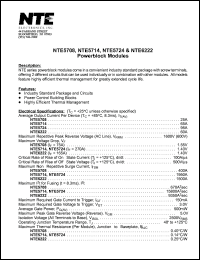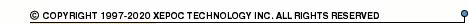More than12 563 416 queries processedNTE622 series datasheets. Manufacturer: NTE.

 NTE5708 Powerblock module. Average output current It(av) = 25A. Max repetitive peak reverse voltage Vrrm = 1600V(600V). in 7-pin -- package. Operational temperature range from -40°C to 125°C. Datasheet*) NTE5714 Powerblock module. Average output current It(av) = 65A. Max repetitive peak reverse voltage Vrrm = 1600V(600V). in 7-pin -- package. Operational temperature range from -40°C to 125°C. Datasheet*) NTE5724 Powerblock module. Average output current It(av) = 95A. Max repetitive peak reverse voltage Vrrm = 1600V(600V). in 7-pin -- package. Operational temperature range from -40°C to 125°C. Datasheet*) NTE6222 Powerblock module. Average output current It(av) = 60A. Max repetitive peak reverse voltage (AC line) Vrrm = 1600V(600V). in 7-pin -- package. Operational temperature range from -40°C to 125°C. Datasheet*) NTE5710 Powerblock module. Average output current It(av) = 55A. Max repetitive peak reverse voltage (AC line) Vrrm = 1200V(480V). in 7-pin -- package. Operational temperature range from -40°C to 125°C. Datasheet*) NTE5711 Powerblock module. Average output current It(av) = 55A. Max repetitive peak reverse voltage (AC line) Vrrm = 1200V(480V). in 5-pin -- package. Operational temperature range from -40°C to 125°C. Datasheet*) NTE5712 Powerblock module. Average output current It(av) = 55A. Max repetitive peak reverse voltage (AC line) Vrrm = 1200V(480V). in 5-pin -- package. Operational temperature range from -40°C to 125°C. Datasheet*) NTE5720 Powerblock module. Average output current It(av) = 90A. Max repetitive peak reverse voltage (AC line) Vrrm = 1200V(480V). in 7-pin -- package. Operational temperature range from -40°C to 125°C. Datasheet*) NTE5721 Powerblock module. Average output current It(av) = 90A. Max repetitive peak reverse voltage (AC line) Vrrm = 1200V(480V). in 5-pin -- package. Operational temperature range from -40°C to 125°C. Datasheet*) NTE5722 Powerblock module. Average output current It(av) = 90A. Max repetitive peak reverse voltage (AC line) Vrrm = 1200V(480V). in 5-pin -- package. Operational temperature range from -40°C to 125°C. Datasheet*) NTE6220 Powerblock module. Average output current It(av) = 55A. Max repetitive peak reverse voltage (AC line) Vrrm = 1200V(480V). in 3-pin -- package. Operational temperature range from -40°C to 125°C. Datasheet*) NTE6230 Powerblock module. Average output current It(av) = 90A. Max repetitive peak reverse voltage (AC line) Vrrm = 1200V(480V). in 3-pin -- package. Operational temperature range from -40°C to 125°C. Datasheet*) NTE622 Silicon rectifier, general purpose, high voltage, fast recovery (surface mount). Max recurrent peak reverse voltage 400V. Max average forward rectified current 0.5A. in 2-pin -- package. Operational temperature range from -65°C to 175°C. Datasheet*)